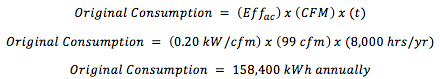# Compressed Air Savings with Nozzles or Blowers

Compressed air is used as a convenient and often necessary source of air flow to perform blow-offs, cooling, or drying.  And since compressed air is a costly utility, a frequent recommendation in this magazine and audits is to reduce the compressed air use by using high efficiency engineered nozzles.  Using these nozzles is a good practice as they are designed in a way that uses the compressed air to accelerate the surrounding air to deliver the same mass transfer effect as a standard nozzle (or tube) with a much larger orifice.

The savings from this switch can be estimated using the orifice sizes and a formula.  While the formula is dependent on temperature and the condition of the inlet air, assuming standard conditions provides the following simplified equation:

CFM = 14.485 x D2 x (psig + 14.7)

Where:

D is the orifice diameter in inches

Psig is the compressed air pressure in pounds per square inch (gauge not absolute)

And CFM is the resulting flow rate in standard cubic feet per minute

This formula can be programmed into a calculator or spreadsheet.  Putting it into a spreadsheet with various pressures and orifice sizes produces the following cfm chart:This can be used to evaluate leaks as well as comparing nozzles.  Screw compressor efficiencies at full load conditions and part load for VSD compressors are typically reported as 18-22 kW per 100 cfm on CAGI data sheets for compressors running at 100 to 120 psi. So conversion from cfm to kW can be done by assuming a compressed air efficiency of about 0.20 kW/cfm.  The amount of energy use (kWh) is then obtained by multiplying by the running hours.

For example, a 1/8” open ended tube at 80 psig would consume 21.4 cfm using the chart.  Multiplying by the compressor efficiency of 0.20 kW/cfm means this would require 4.28 kW input to the compressor.  If this runs 6000 hrs/yr it would use 25,680 kWh and cost \$2,568 at an electric cost of \$0.10/kWh.  Replacing this with a high efficiency nozzle rated to use 13 cfm at 80 psi would save 8.4 cfm, 1.68 kW, 10,080 kWh, and \$1,000.  With a nozzle cost of about \$50 this would be very attractive.  This same type of analysis can also be used to assign a monetary value to air leaks.

### Blowers

The ideal approach to optimize energy use is to not use compressed air at all by using a blower to provide the low pressure air flow.  This has a much higher capital cost (and effort) except in cases where it would avoid the purchase of an air compressor.  The savings analysis evaluates the cost of the current compressed air use by the nozzle or open pipe system as shown above, and then evaluates the cost of running the motor on the blower to obtain the difference.

For example, one site that manufactured metal parts for the automotive industry washed machining oils off the parts, and then proceeded to dry the parts using two air amplifiers at a line pressure of 90-100 psi.  The wash line is a continuous conveyor holding a steady line of parts.  This kept the blow-off running continuously.  A flow meter installed on the line showed the flow rate to be 99 scfm.  The plant chose to install a 15 hp blower rated at 800 cfm and then set the blower speed as needed with a VFD.  The plant normally operates 8,000 hrs/yr.  While this also increased volume capacity, it still demonstrated energy savings.  Original energy use would be the following:In this particular case post monitoring was performed by data logging the power use of the blower.  The inverter frequency was set at 60 Hz.  Staff recognized it would be possible to run at lower rates but had not yet attempted to do so.  The power demand was steady at 8.8 kW.  The new energy use is thus the 8.8 kW demand multiplied by the 8,000 hour run time which is 70,400 kWh.

The annual energy savings is the original consumption of 158,400 kWh less the new use of 70,400 kWh which is 88,000 kWh.  This site has an average electricity cost of \$0.08/kWh so the annual savings are \$7,040.  This qualified for an energy efficiency rebate of \$7,000, and the full project cost was reported to be \$23,500 including blower, inverter, hoses, enclosure, and installation.  This project provided a simple payback of 2.3 years after rebate.

Blow-off applications using compressed air are common, and the compressed air used for them is costly.  Many of these systems can use less compressed air through the use of high efficiency nozzles or blowers.  High efficiency nozzles are typically easy and inexpensive to implement by adding a nozzle where there is presently a standard nozzle or an open tube, and the savings can be significant.  The compressed air use can be eliminated entirely in some cases by using a blower.  This offers greater savings than compressed air nozzles and preserves system capacity, but it requires effort and expense to implement.